Learn All Concepts of Chapter 1 Class 9 - FREE. Check - Chapter 1 Class 9 Maths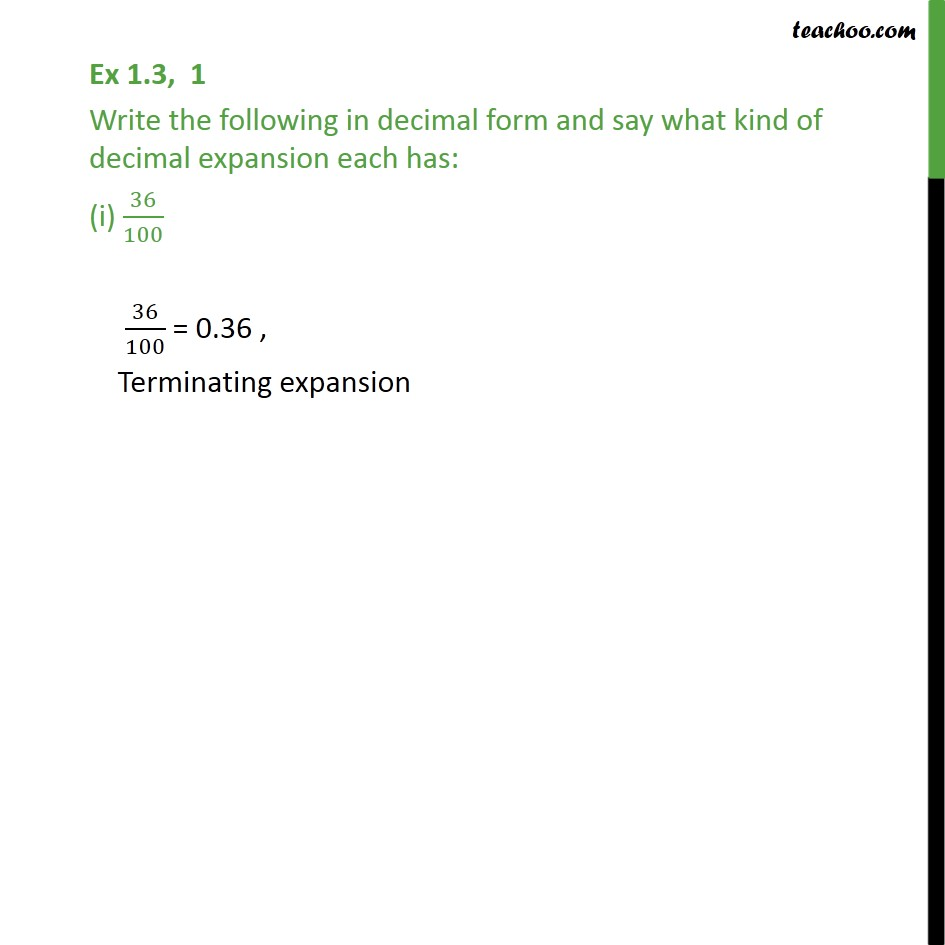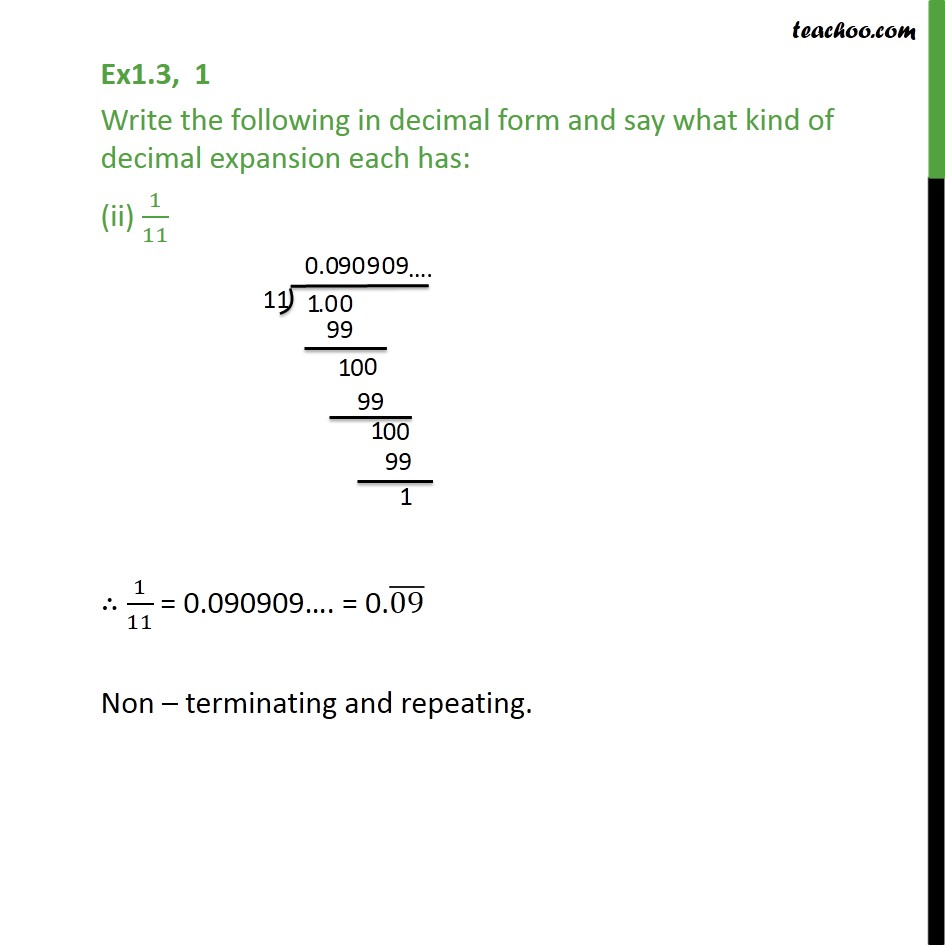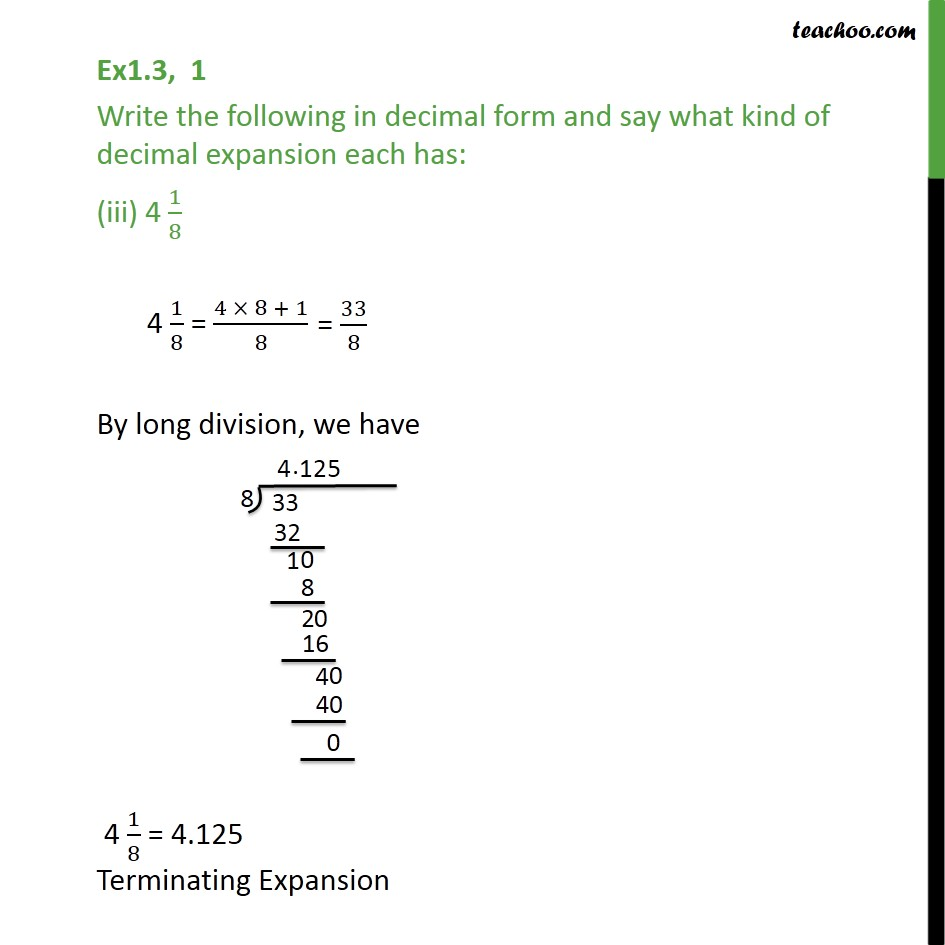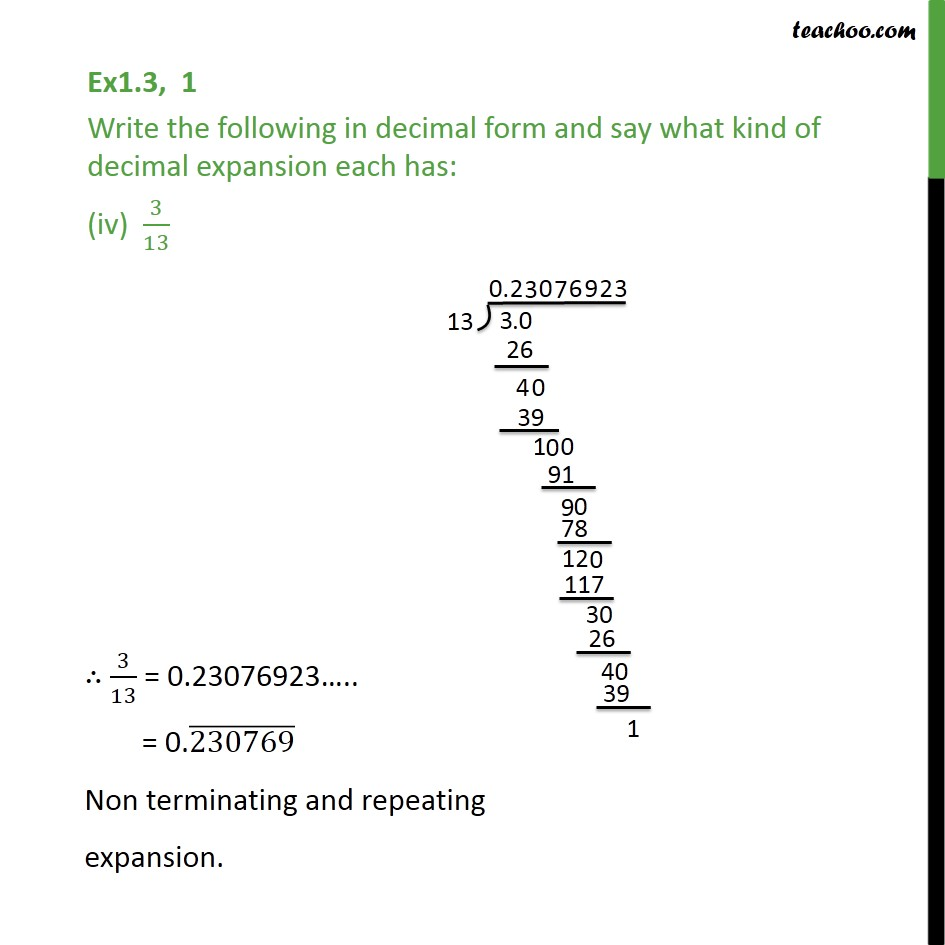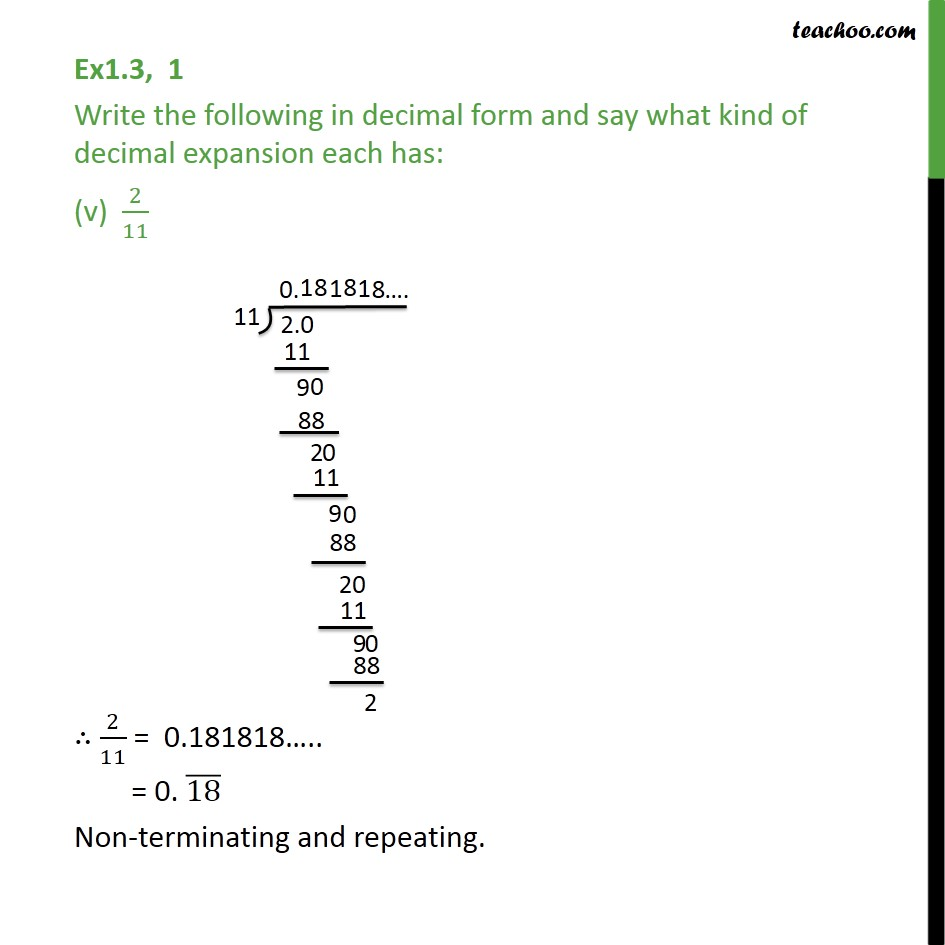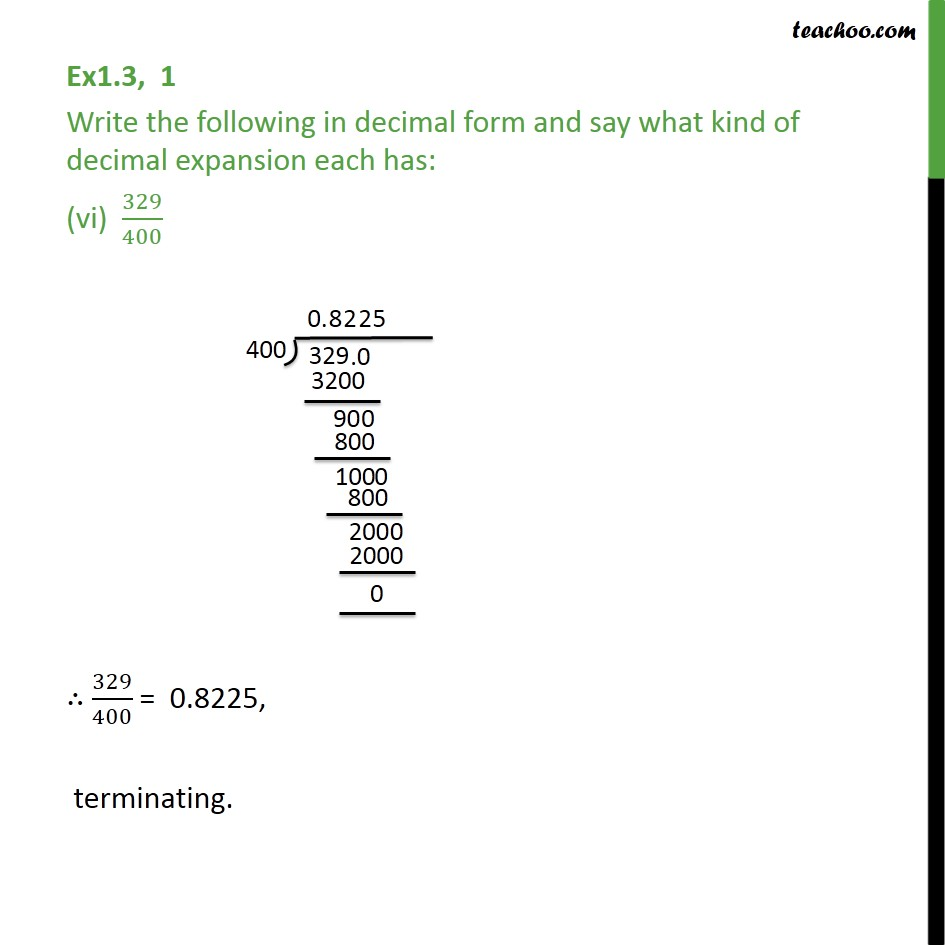1. Chapter 1 Class 9 Number Systems
2. Serial order wise
3. Ex 1.3

Transcript

Ex 1.3, 1 Write the following in decimal form and say what kind of decimal expansion each has: (i) 36/100 36/100 = 0.36 , Terminating expansion Ex1.3, 1 Write the following in decimal form and say what kind of decimal expansion each has: (ii) 1/11 ∴ 1/11 = 0.090909…. = 0.(09) ̅ Non – terminating and repeating. Ex1.3, 1 Write the following in decimal form and say what kind of decimal expansion each has: (iii) 4 1/8 4 1/8 = (4 × 8 + 1)/8 By long division, we have Ex1.3, 1 Write the following in decimal form and say what kind of decimal expansion each has: (iv) 3/13 Ex1.3, 1 Write the following in decimal form and say what kind of decimal expansion each has: (v) 2/11 ∴ 2/11 = 0.181818….. = 0. (18) ̅ Non-terminating and repeating. Ex1.3, 1 Write the following in decimal form and say what kind of decimal expansion each has: (vi) 329/400 ∴ 329/400 = 0.8225, terminating.

Ex 1.3